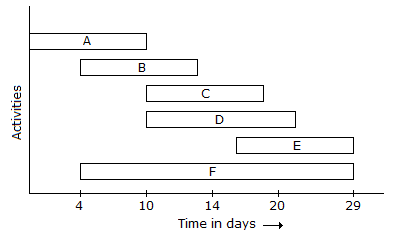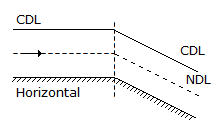# Civil Engineering - UPSC Civil Service Exam Questions

41.

Which are the critical activities of the bar chart shown above ?A. Activities B and E B. Activities A, D and F C. Activities A, C and E D. Activities A and F

Explanation:

No answer description available for this question. Let us discuss.

42.

What is the effective height of a free-standing masonry wall for the purpose of computing slenderness ratio ?

 A. 0.5 L B. 1.0 L C. 2.0 L D. 2.5 L

Explanation:

No answer description available for this question. Let us discuss.

43.

To generate 10, 000 hp under a head of 81 m while working at a speed of 500 rpirt, the turbine of choice would be

 A. Pelton B. Kaplan C. Bulb D. Francis

Explanation:

No answer description available for this question. Let us discuss.

44.

Consider the following statements :
Sand in mortar is needed for
1. decreasing the quantity of cement.
2. reducing shrinkage.
3. decreasing the surface area of the binding material.
4. increasing the strength.
Of these statements :

 A. 2, 3 and 4 are correct B. 1, 2 and 3 are correct C. 1, 3 and 4 are correct D. 1, 2 and 4 are correct

Explanation:

No answer description available for this question. Let us discuss.

45.

The given figure shows gradually varied flow in an open channel with a beak in bed slope. Types of water surface profiles occurring from left to right areA. H2 , S3 B. H2 , S2 C. H2 , M2 D. H3 , M2